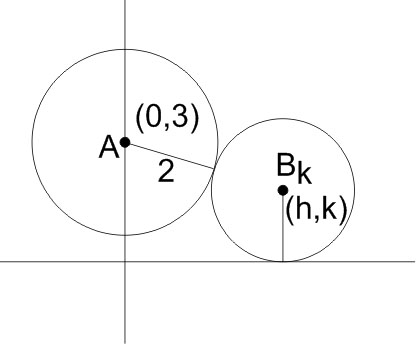#### A circles touches theaxis and also touches the circle with centre at (0, 3) and radius 2. The locus of the centre of the circle is Option 1) a circle Option 2) an ellipse Option 3) a parabola Option 4) a hyperbola.As we learnt in

Circle touching x-axis and having radius r -- wherein

Where f is a variable parameter.

General form of a circle -- wherein

centre =radius =Let centre of circle be P(h,k)

distance  AB =Squaring both sidesWhich represents a parabola.

Option 1)

a circle

Incorrect option

Option 2)

an ellipse

Incorrect option

Option 3)

a parabola

Correct option

Option 4)

a hyperbola.

Incorrect option

#### Plabita